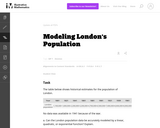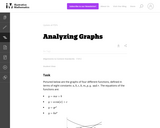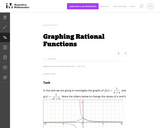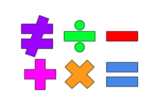Updating search results...

# 4 Results

View
Selected filters:
• UT.MATH.III.F.IF.7Unrestricted Use
CC BY
Rating
0.0 stars

This is a task from the Illustrative Mathematics website that is one part of a complete illustration of the standard to which it is aligned. Each task has at least one solution and some commentary that addresses important asects of the task and its potential use. Here are the first few lines of the commentary for this task: The table below shows historical estimates for the population of London. Year18011821 18411861 18811901 1921 1939 1961 London population 1,100,000 1,60...

Subject:
Mathematics
Secondary Mathematics
Material Type:
Activity/Lab
Provider:
Illustrative Mathematics
Provider Set:
Illustrative Mathematics
Author:
Illustrative Mathematics
10/30/2013Unrestricted Use
CC BY
Rating
0.0 stars

This is a task from the Illustrative Mathematics website that is one part of a complete illustration of the standard to which it is aligned. Each task has at least one solution and some commentary that addresses important asects of the task and its potential use. Here are the first few lines of the commentary for this task: Pictured below are the graphs of four different functions, defined in terms of eight constants: $a, b, c, k, m, p, q, \text{ and } r.$ The equations of...

Subject:
Mathematics
Material Type:
Activity/Lab
Provider:
Illustrative Mathematics
Provider Set:
Illustrative Mathematics
Author:
Illustrative Mathematics
08/20/2013Unrestricted Use
CC BY
Rating
0.0 stars

This is a task from the Illustrative Mathematics website that is one part of a complete illustration of the standard to which it is aligned. Each task has at least one solution and some commentary that addresses important asects of the task and its potential use. Here are the first few lines of the commentary for this task: In this task we are going to investigate the graphs of $\displaystyle{f(x) = \frac{1}{x+a}}$ and $\displaystyle{g(x) = \frac{1}{x^2+b}}.$ Move the slid...

Subject:
Mathematics
Secondary Mathematics
Material Type:
Activity/Lab
Provider:
Illustrative Mathematics
Provider Set:
Illustrative Mathematics
Author:
Illustrative Mathematics
11/25/2013Unrestricted Use
Public Domain
Rating
0.0 stars

This lesson is for a math classroom, but can be adapted to fit any grade, subject, or content.&nbsp;&nbsp;In this lesson, students will use an iPad and its features: Keynote, Pages, Garageband, Numbers, Presentation, and iMovie.&nbsp; Students will use 3 of those features/programs to create a video lesson consisting of several examples from a topic of their choice.&nbsp; This project is in place of a term final, so their chosen topic should be from their current term.Image citation: The image is one I created.&nbsp;&nbsp;

Subject:
Secondary Mathematics
Material Type:
Assessment
Author:
Tyrani Bevell